# How to Format Numbers to Thousands & Millions & Billions in Excel

This post will guide you how to format numbers into thousands or millions or billions with Format Cells function in Excel 2013/2016. How do I format large numbers with Thousands or Millions separators in Excel.

For example, number 8000 should be shown as 8 or 8K, 8000000 should be shown as 8 or 8M. and Excel does not provide such option with a single click or way. And you can use number formatting feature to achieve the result.

Excel Number Formatting is a larger feature in Excel, and we have written lots of posts which includes all kinds of number formatting in Excel. Number Formatting allows you to modify the appearance of cell values without changing their real values. Also you can also add Thousands or Millions separators without changing the cell actual values.

In Microsoft Excel, if you want to improve the readability of your data by formatting numbers to show as thousands(k), Millions(M), or Billions(B). The below steps will show you how to format numbers with Thousands or Millions separators to show in a shorter format to read and understand very easily by creating a custom number format.

## Format Numbers in Thousands

Firstly, we will show you how to format numbers in thousands by create a custom format with the Format Cells function in your worksheet.

Assuming that you have a list of data with the below set of numbers in cell range A1:A5. And now we need to format these numbers in thousands.

Just do the following steps to change the formatting of the numbers:

Step1: select your numbers in range A1:A5.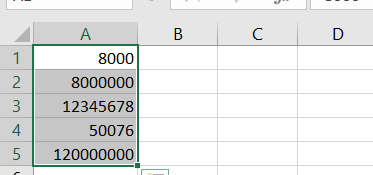Step2: right click on the selected cells that you want to format. and select Format Cells menu from the pop-up menu list. And the Format Cell dialog box will appear. Or you can also press the shortcut key CTRL +1 to open the Format Cells dialog box.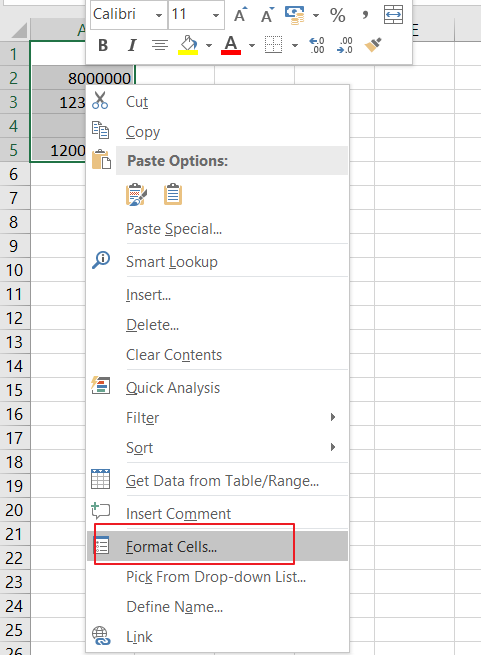Step3: click Number tab in the Format Cells dialog box, and click the Custom option from the left pane.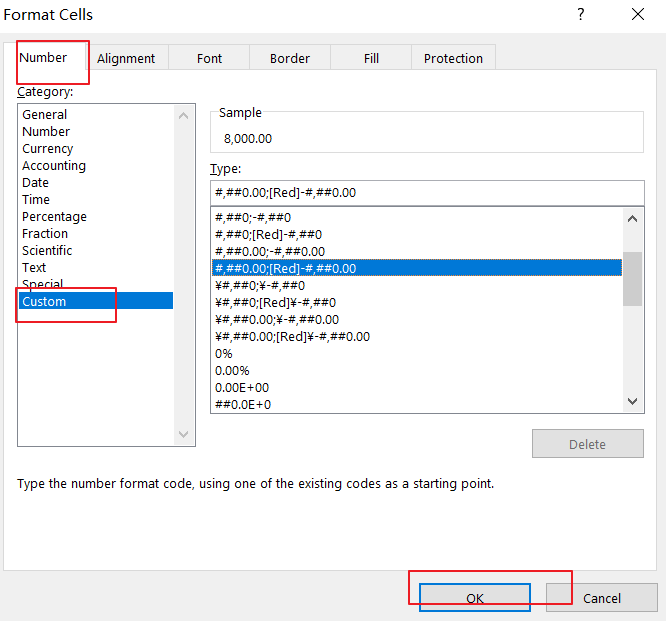Step4: now go to Type: section in the Format Cells dialog box, add the following formatting code to change the formatting of the selected numbers. Click Ok button.

`0, "K"`

Or

`0, “ Thousands”`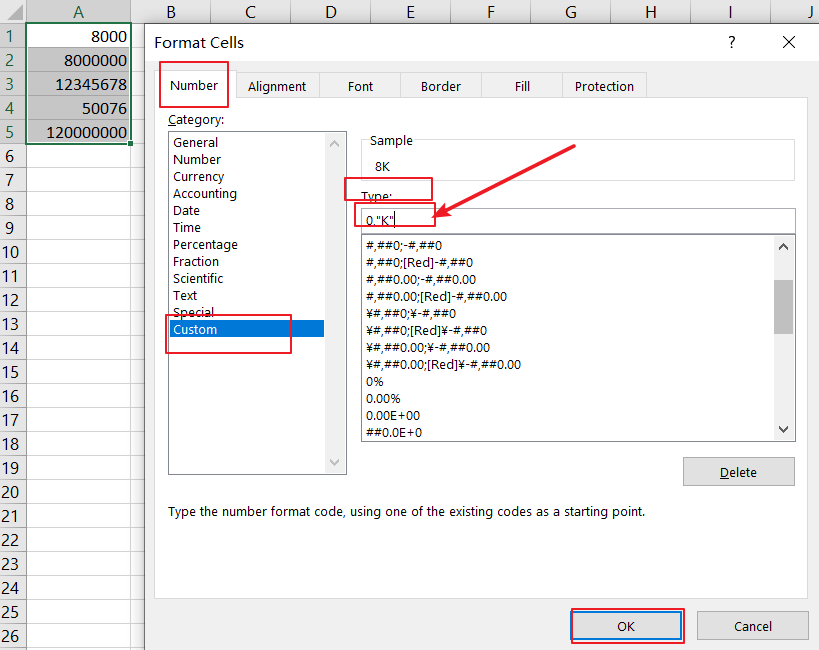Step5: your selected numbers will appear in thousands automatically. And the formatting does not change the integrity or truncate your numeric values in any way. And it will apply a cosmetic effect to the number.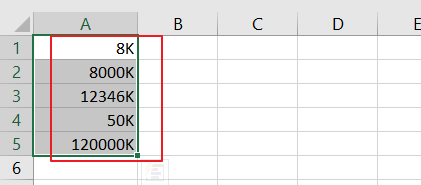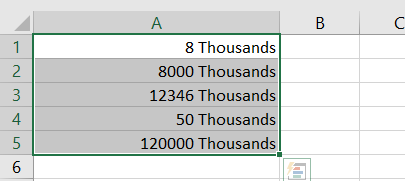If you want to show the exact value, and you can change the formatting code as below:

`0.000,”K”`

Or

`0.000,” Thousands”`

Then you will see that the exact values with decimal points should be shown.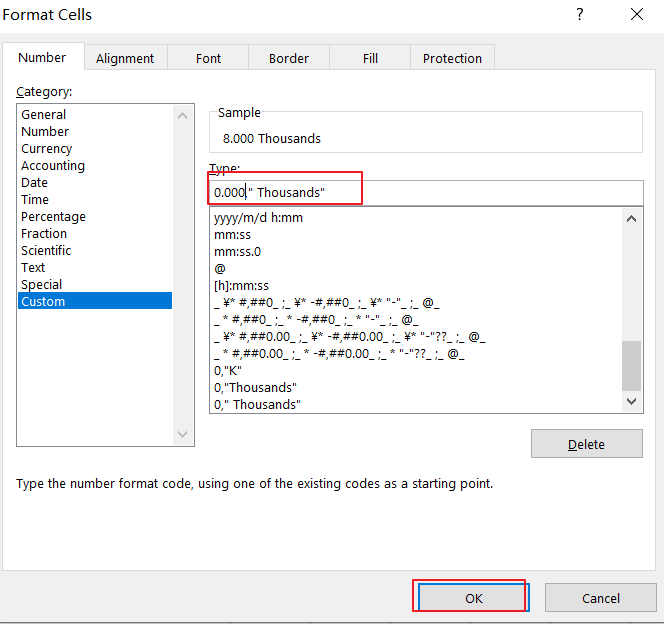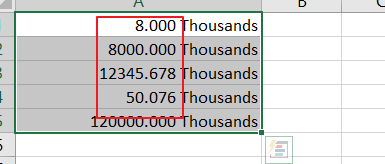## Format Numbers in Thousands with Formula

There is another method to format numbers in thousands separator in Excel. And you can create a formula based on concentrate character. You need to divide the number by 1000 and combine the word “Thousands” or character “K” by using concentrate character “&”. Type the following formula in a blank cell:

`=A1/1000 & “K”`

Or

`=A1/1000 & “ Thousand”`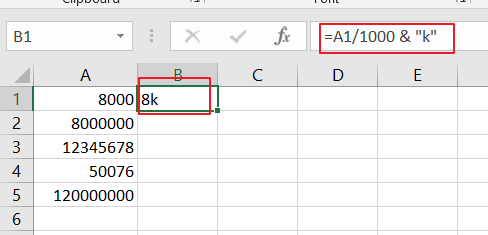Then you can drag the Fill Handle down to other cells to apply this formula.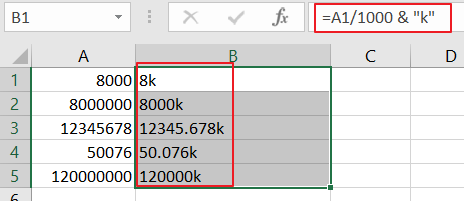## Format Numbers in Millions

The above steps have shown you how to format numbers in thousands, and the below steps will show you how to format number in Millions. Just do the following steps:

Step1: open the Format Cells dialog box, and click the Custom option.

Step2: add the following format code in the Type: section. The only difference between previous code and this format code is that you need to add one extra comma(,).

`0.000,, “M”`

Or

`0.000,, “ Million”`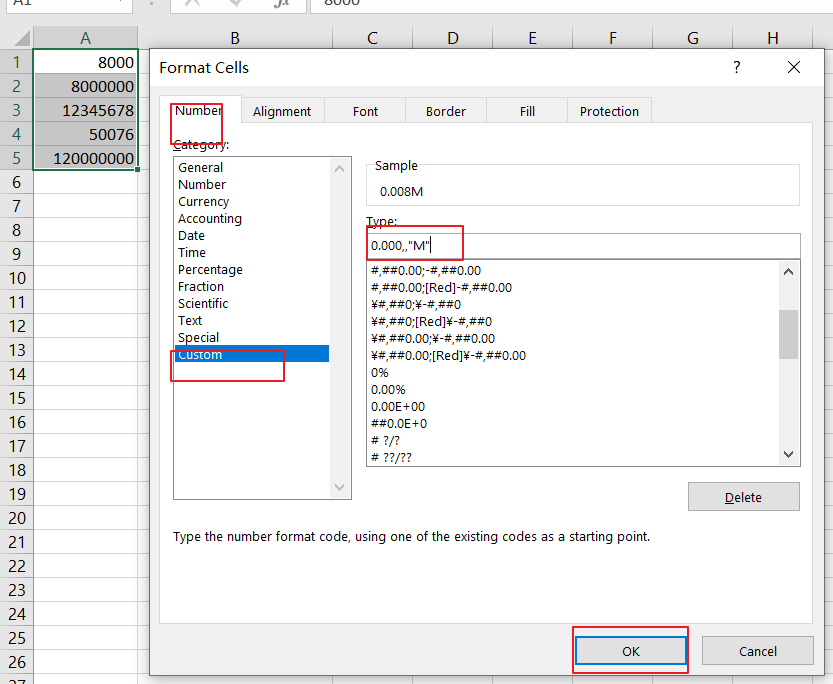Step3: the result is as below: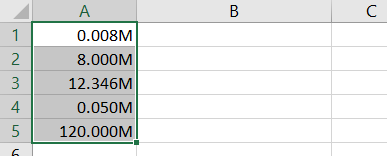## Format Numbers in Billions

In the previous step we have talked that how to format numbers in thousands and millions. And now we will see that how to format numbers in Billions.

You just need to refer to the above steps, and then use another format code to change the formatting of the numbers in Billions.

`0.000,,, “B”`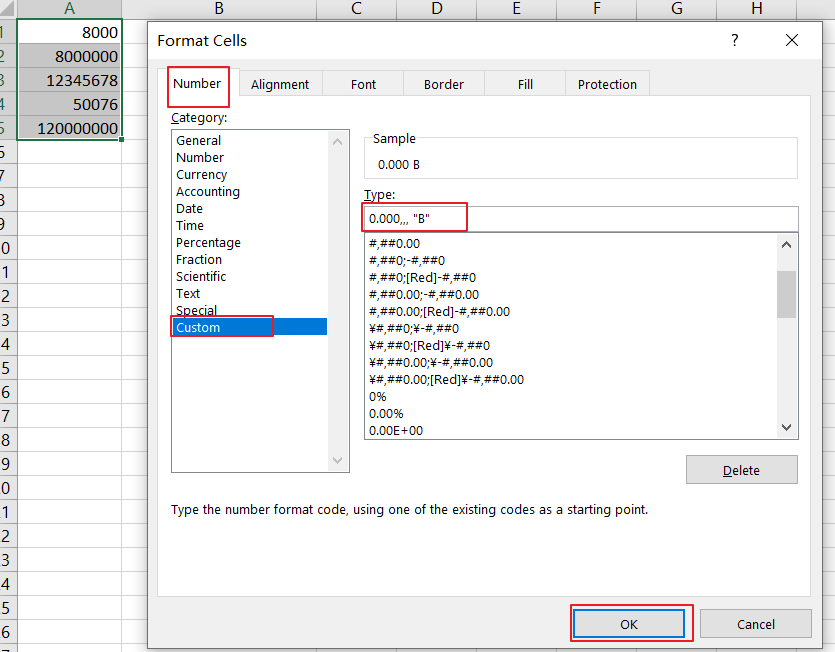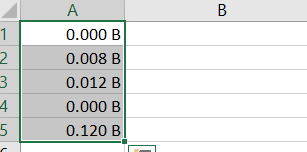## Format Numbers in Thousands, Millions, Billions Based on Numbers

If you want to format numbers to show the result based on the cell values. For example, if the cell value is less than 10000, and the result display as 10K, and if the cell value is greater than or equal to 1000000, then the result is displayed in Million. You can add the following format code into Type: section.

`[<999950]0.0,"K";[<999950000]0.0,,"M";0.0,,,"B"`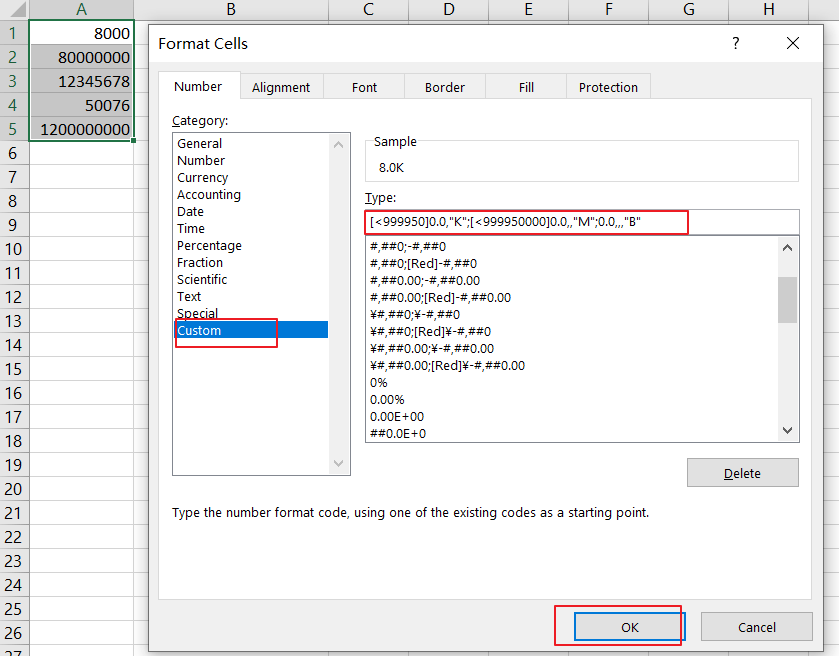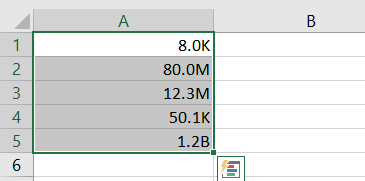Related Posts

Cap percentage values between 0 and 100

This article will talk about how to cap the percentage values between 0% and 100% in Microsoft Excel Spreadsheet or Google Sheets. If you are a newbie on Excel or google Sheets, you may be able to do this by ...

Calculate Cap Percentages to Specific Value

This article will talk about how to limit the cap percentage of a given amount to a given value in Microsoft Excel Spreadsheet or Google Sheets. If you are a newbie on Excel or google Sheets, you may be able ...

Calculate Win Loss Tie

Suppose you got a task to calculate the win, loss, and tie totals; what would you do? If you are new to Ms Excel and don't have enough experience with it, then you might do this task manually but let ...

Calculate Years Between Dates In Ms Excel

If you are an avid Ms Excel user, then you might have come across a task in which you needed to calculate the years between the dates; you might take it easy and do this task manually, which is also ...

Calculate Number of Hours between Two Times

Calculating the difference between two times might be a valuable statistic for subsequent computations or averages, whether you're producing a time sheet for staff or recording personal exercises. While Excel has a plethora of complex functions, including date and time ...

Calculate Loan Interest in Given Year

When you borrow money, you are supposed to repay it gradually. Lenders, on the other hand, want to be compensated for their services and the risk they incur by lending you money. That is, you will not just repay the ...

Calculate Interest Rate for Loan

The interest rate is the fee charged by a lender to a borrower and is expressed as a percentage of the principal—the lent amount. The interest rate on a loan is often expressed as an annual percentage rate, abbreviated as ...

Calculate Interest for Given Period

Using the IPMT function in Excel, we can compute the interest payment on any loan. This step-by-step tutorial will guide Excel users of all skill levels through the process to calculate interest for given period. Finally, the formula: =IPMT(B3/12,1,B5,-B2) The ...

How To Use Excel GCD Function

This post will guide you how to use Excel GCD function with syntax and examples in Microsoft excel. Description The Excel GCD function Returns the greatest common divisor of two or more integers. So you can use the GCD function ...

Calculate A Ratio From Two Numbers In Excel

In elementary mathematics, a ratio is a connection or comparison between two or more integers. For example, ratios are often expressed as ":" to demonstrate the relationship between two numbers. You would think that manually calculating a ratio from two ...

Sidebar